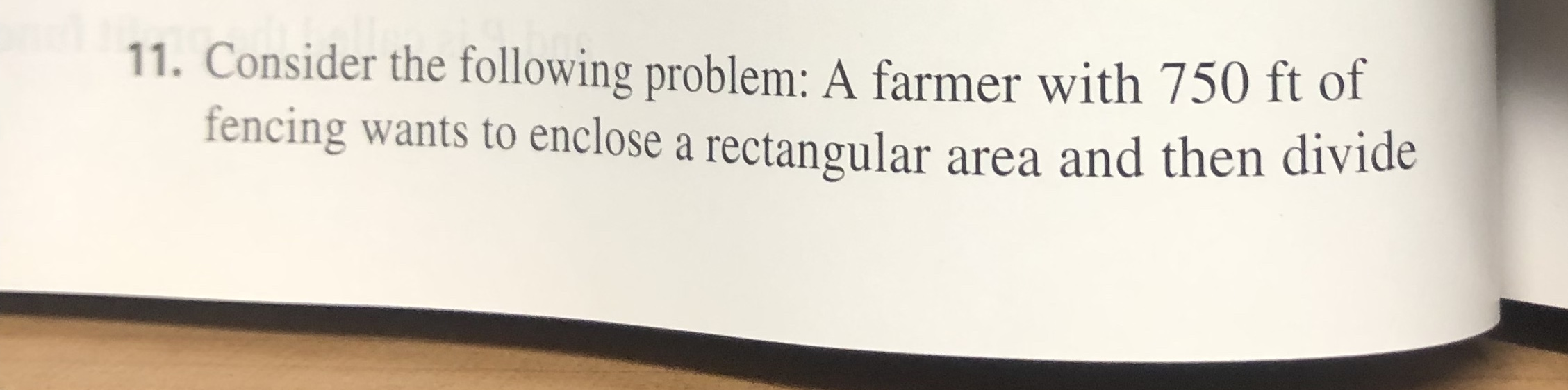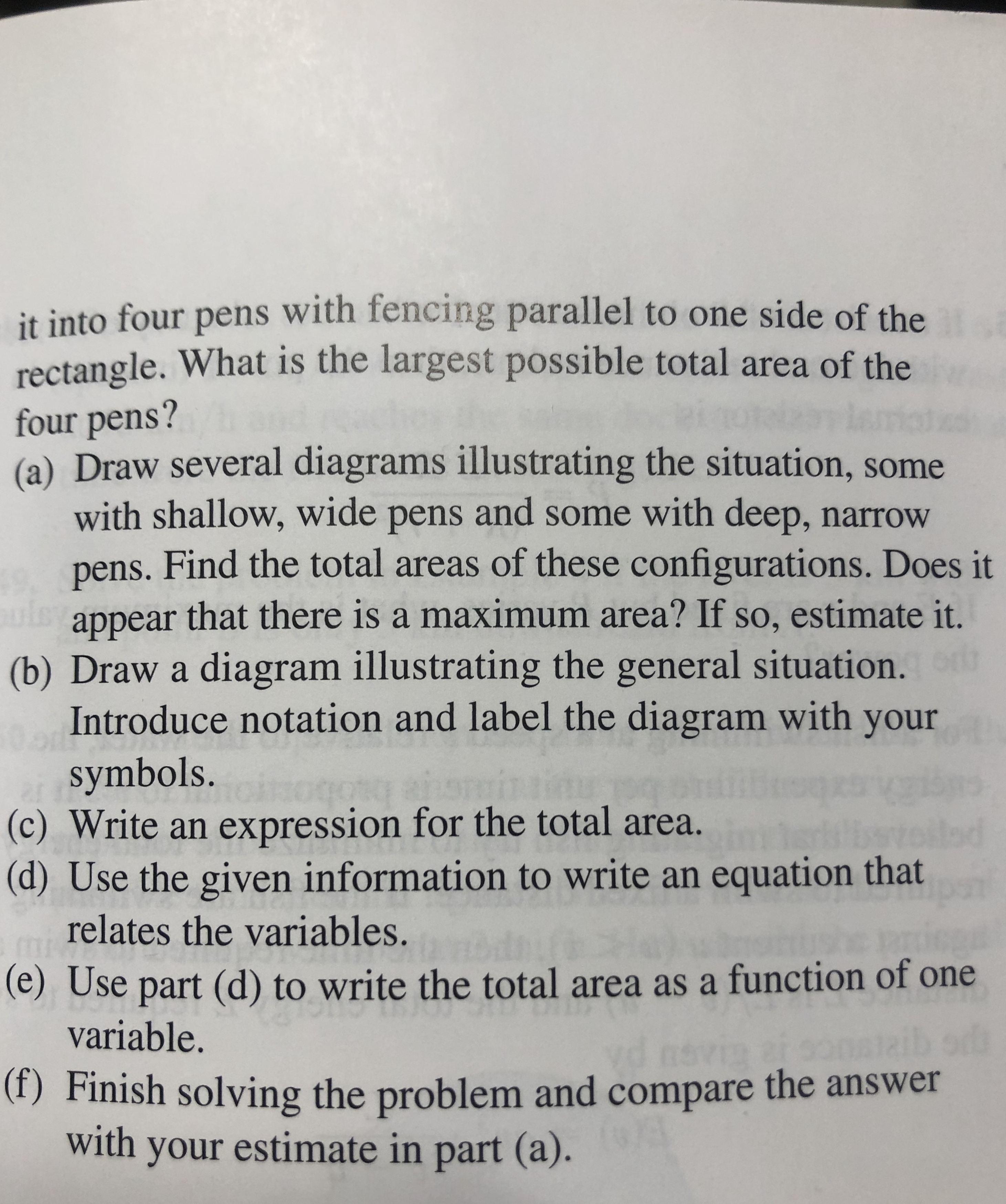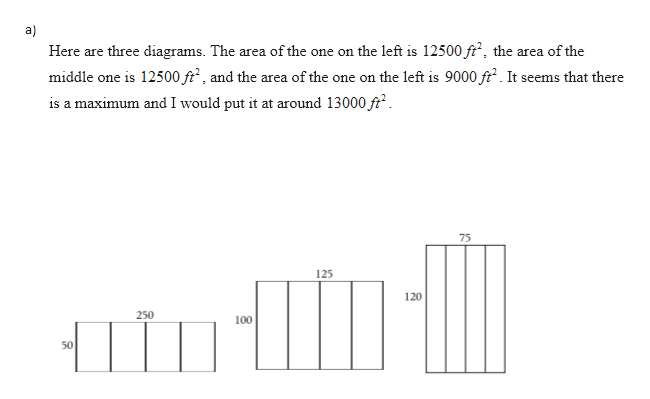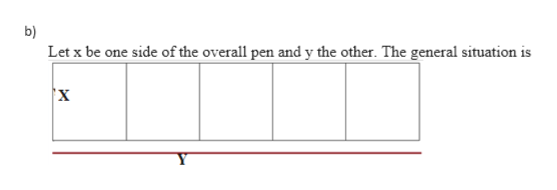# 11. Consider the following problem: A farmer with 750 ft offencing wants to enclose a rectangular area and then divide it into four pens with fencing parallel to one side of therectangle. What is the largest possible total area of thefour pens?(a) Draw several diagrams illustrating the situation, somewith shallow, wide pens and some with deep, narrowpens. Find the total areas of these configurations. Does itappear that there is a maximum area? If so, estimate it.(b) Draw a diagram illustrating the general situation.Introduce notation and label the diagram with yoursymbols.(c) Write an expression for the total area.(d) Use the given information to write an equation thatrelates the variables.(e) Use part (d) to write the total area as a function of onevariable.alaibod(f) Finish solving the problem and compare the answerwith your estimate in part (a).

Question
34 views

3.7

Can you show me all the steps to solve this problem?help_outlineImage Transcriptionclose11. Consider the following problem: A farmer with 750 ft of fencing wants to enclose a rectangular area and then divide fullscreenhelp_outlineImage Transcriptioncloseit into four pens with fencing parallel to one side of the rectangle. What is the largest possible total area of the four pens? (a) Draw several diagrams illustrating the situation, some with shallow, wide pens and some with deep, narrow pens. Find the total areas of these configurations. Does it appear that there is a maximum area? If so, estimate it. (b) Draw a diagram illustrating the general situation. Introduce notation and label the diagram with your symbols. (c) Write an expression for the total area. (d) Use the given information to write an equation that relates the variables. (e) Use part (d) to write the total area as a function of one variable. alaibod (f) Finish solving the problem and compare the answer with your estimate in part (a). fullscreen
check_circle

Step 1

Given that

Length of fencing is 750 ft

Step 2help_outlineImage Transcriptionclosea) Here are three diagrams. The area of the one on the left is 12500 ft2, the area of the middle one is 12500 ft2, and the area of the one on the left is 9000 ft2. It seems that there is a maximum and I would put it at around 13000 ft2 75 125 120 250 100 50 fullscreen
Step 3help_outlineImage Transcriptioncloseb) Let x be one side of the overall pen and y the other. The general situation is 'х fullscreen

### Want to see the full answer?

See Solution

#### Want to see this answer and more?

Solutions are written by subject experts who are available 24/7. Questions are typically answered within 1 hour.*

See Solution
*Response times may vary by subject and question.
Tagged in
MathCalculus

### Derivative